## Simple Populations: n=2, l=2

We will experiment with the smallest sets possible: n=2 (number of individuals in a population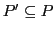), l=2 (number of positions in an individual, binary format). From this follows, that there are maximal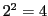many different patterns, that means that the world of possible events, the maximal populationhas 4 possible individuals. Because we assume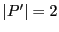we have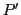as a true subset of.

``` -->P=[0 0; 0 1; 1 0; 1 1]
P  =

0.    0.
0.    1.
1.    0.
1.    1.
```

The probabilities for this population are given in the following figure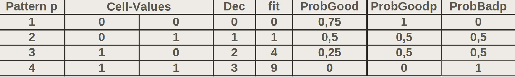From this we can get subsetslike POP1={0 0; 0 1}, POP2={0 1; 1 0}, POP3={0 1; 1 1} etc. Translating the binary numbers in decimal numbers and applying the fitnes function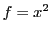we can infer that the maximal possible value of a populationis given with the subset {1 1; 1 1} as the sum of the individual fitness values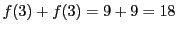. Thus a populationwith the sum of all fitness values as '18' would represent 100%. All others would be 'below' 100%.

Knowing this one can investigate the 'behavior' of such populations under crossover and mutation.

``` POP1  =

0.    0.
0.    1.
```

Then we use POP as temporary buffer:

``` -->POP=POP1
POP  =

0.    0.
0.    1.
```

Then we call the function gasimple() to compute some cycles with crossover and selection but without mutation (we are using the script GA_v5.sce)(One can also use GA_v4.sce).

``` -->n=2, l=2, p=5, run=20, MThreshold=20,[POP,FITNESS_ALLLOG]=gasimple(POP,l,p,n,run, MThreshold)
```

All the data are arranged in a matrix with the first l-many elements for the members of the population and the next p-many elements for some additional parameters. Because the MThreshold-parameter is as high as the runs-parameter there will no mutation happen.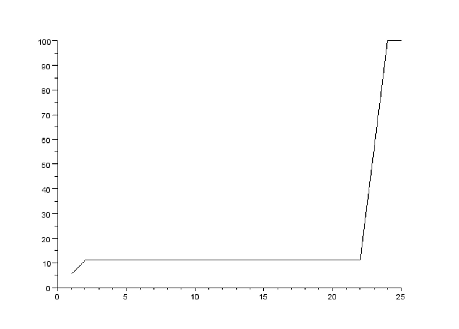As one can see without mutation (cycles 1-19) the maximal value of '2' (= 11 %) will be reached in two cycles, then nothing further will happen until a mutation is allowed at cycle 20 (MThreshold=20). This induces a big shift until the value '18' (= 100 %).

In the following example with POP2 you can observe three different cases: (i) It needs some time to find the maximum (as depicted in figure 5.4); (ii) After reaching a maximum the values can decrease again because the probability to find a new better value is zero, but the probability to find something more 'worse' is 100% (cf. figure 5.5); (iii) generally one has to assume a cyclic appearance of the population values because the propability for 'goog' and 'bad' values is compementary. As far one kind of values has a majority then the other kind gets a higher probability (cf. 5.6).

```-->POP2=[0 1; 1 0]
POP2  =

0.    1.
1.    0.

-->POP=POP2
POP  =

0.    1.
1.    0.
```

```-->n=2, l=2, p=5, run=200,MThreshold=15,[POP,FITNESS_ALLLOG]=gasimple(POP,l,p,n,run, MThreshold)
```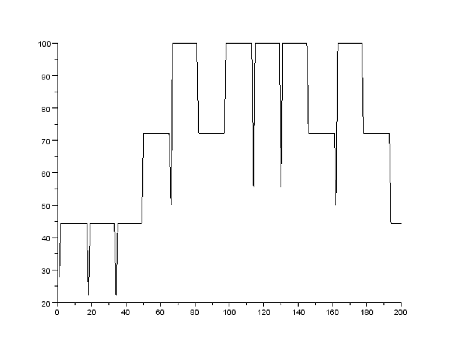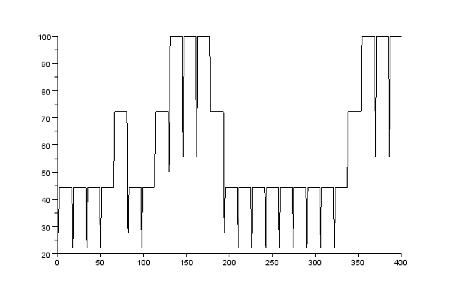The last example with these simple populations shows a populationwith nearly optimal values. According to the population dynamics implies this a 100% pobability to find by mutation 'weaker' population values which represents a decrease in the overall values (cf. figure 5.7).

```-->POP3=[0 1; 1 1]
POP3  =

0.    1.
1.    1.

-->POP=POP3
POP  =

0.    1.
1.    1.
```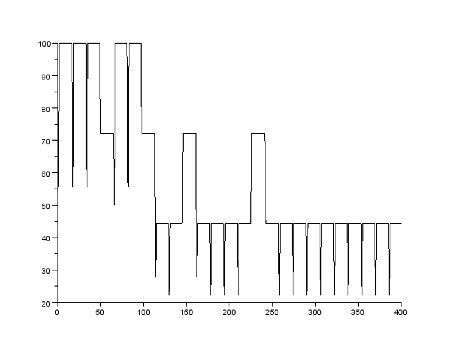These examples underline the general theoretical issue that crossover does not matter while mutation can help as long as the population has not yet reached it's maximum value. As closer is a population as lower is the probability to find a better value and the inverse probability increases to find a value more worse.

Gerd Doeben-Henisch 2013-01-14